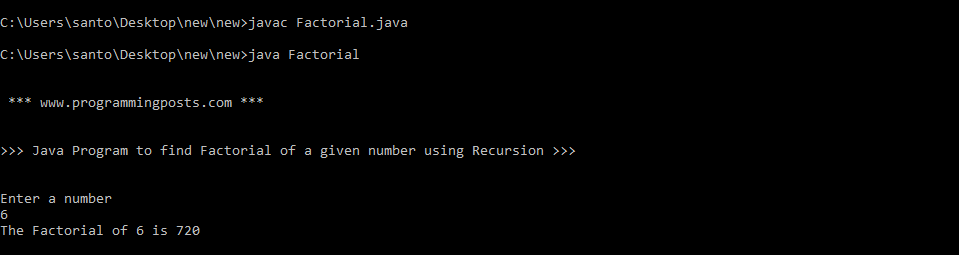# Java program to find Factorial of a given number using Recursion

### Factorial:

Factorial of a non-negative integer n, is the product of all positive integers less than or equal to n.  It is represented as n!.

n! = 1 * 2 * 3 * 4 * ………………… * (n-1) * n.

for example,  5! can be calculated as 5 * 4 * 3* 2 * 1 = 120.

### Recursion:

Recursion  is a process in which a method calls itself continuously.  A method in java that calls itself is called recursive method.

For example a factorial can be defined recursively as:

factorial(x) = x * factorial(x – 1)  for x > 1
= 1 for x = 1 or x = 0

The base criteria for the function is at x = 1 or x = 0 .i.e. the function does not call itself when it reaches x = 1 or x = 0.The function calls itself whenever x is not 1 or 0 and moves closer to the base criteria as x is reduced at every recursive call.

### Algorithm:

Step 1: START.Step 2: Read number n.

Step 3: call  factorial(n)

Step 4: print factorial f.

Step 5: stop.

factorial(n)

Step 1: If n==1  then return 1

Step 2: Else  f=n*factorial(n-1)

Step 3: Return f

### Source code:

```import java.util.*;
class Factorial
{
static int factorial(int num)
{
if(num == 0)
{
return 1;
}
else
{
int fact;
fact = num * factorial(num-1);
return fact;
}
}
public static void main(String args[])
{
System.out.println("\n \n *** www.programmingposts.com *** \n \n");
System.out.println(">>> Java Program to find Factorial of a given number using Recursion >>> \n\n ");
int fact = 1;
Scanner scanner = new Scanner(System.in);
System.out.println("Enter a number");
int num = scanner.nextInt();
fact = factorial(num);
System.out.println("The Factorial of " + num + " is " +fact);
}
}```

### Explanation:

The factorial of a number be found using recursion also. The base case can be taken as the factorial of the number 0 or 1, both of which are 1. The factorial of some number num is that number multiplied by the factorial of (num-1). Mathematically,

factorial ( 0 ) = 1
factorial ( num ) = n * factorial ( num – 1 ).
where num is taken as the input from the user.
The factorial() is called from the main() with the num is passed as an argument.

### Output:

C:\Users\santo\Desktop\new\new>javac Factorial.javaC:\Users\santo\Desktop\new\new>java Factorial

*** www.programmingposts.com ***

>>> Java Program to find Factorial of a given number using Recursion >>>

Enter a number
6
The Factorial of 6 is 720.

### Screenshot of output:Screenshot of output